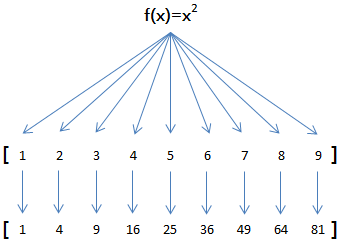> Python3中文手册 > 高阶函数

高阶函数

变量可以指向函数

Python内置的求绝对值的函数abs()为例，调用该函数用以下代码：

>>> abs(-10)
10

>>> abs
<built-in function abs>

>>> x = abs(-10)
>>> x
10

>>> f = abs
>>> f
<built-in function abs>

>>> f = abs
>>> f(-10)
10

函数名也是变量

>>> abs = 10
>>> abs(-10)
Traceback (most recent call last):
File "<stdin>", line 1, in <module>
TypeError: 'int' object is not callable

abs指向10后，就无法通过abs(-10)调用该函数了！因为abs这个变量已经不指向求绝对值函数而是指向一个整数10

传入函数

return f(x) + f(y)

x = -5
y = 6
f = abs
f(x) + f(y) ==> abs(-5) + abs(6) ==> 11
return 11

11

小结

Python内建了map()reduce()函数。>>> def f(x):
...     return x * x
...
>>> r = map(f, [1, 2, 3, 4, 5, 6, 7, 8, 9])
>>> list(r)
[1, 4, 9, 16, 25, 36, 49, 64, 81]

map()传入的第一个参数是f，即函数对象本身。由于结果r是一个IteratorIterator是惰性序列，因此通过list()函数让它把整个序列都计算出来并返回一个list。

L = []
for n in [1, 2, 3, 4, 5, 6, 7, 8, 9]:
L.append(f(n))
print(L)

>>> list(map(str, [1, 2, 3, 4, 5, 6, 7, 8, 9]))
['1', '2', '3', '4', '5', '6', '7', '8', '9']

reduce(f, [x1, x2, x3, x4]) = f(f(f(x1, x2), x3), x4)

>>> from functools import reduce
...     return x + y
...
>>> reduce(add, [1, 3, 5, 7, 9])
25

>>> from functools import reduce
>>> def fn(x, y):
...     return x * 10 + y
...
>>> reduce(fn, [1, 3, 5, 7, 9])
13579

>>> from functools import reduce
>>> def fn(x, y):
...     return x * 10 + y
...
>>> def char2num(s):
...     return {'0': 0, '1': 1, '2': 2, '3': 3, '4': 4, '5': 5, '6': 6, '7': 7, '8': 8, '9': 9}[s]
...
>>> reduce(fn, map(char2num, '13579'))
13579

from functools import reduce

def str2int(s):
def fn(x, y):
return x * 10 + y
def char2num(s):
return {'0': 0, '1': 1, '2': 2, '3': 3, '4': 4, '5': 5, '6': 6, '7': 7, '8': 8, '9': 9}[s]
return reduce(fn, map(char2num, s))

from functools import reduce

def char2num(s):
return {'0': 0, '1': 1, '2': 2, '3': 3, '4': 4, '5': 5, '6': 6, '7': 7, '8': 8, '9': 9}[s]

def str2int(s):
return reduce(lambda x, y: x * 10 + y, map(char2num, s))

lambda函数的用法在后面介绍。

参考代码

do_map.py

do_reduce.py

Python内建的filter()函数用于过滤序列。

map()类似，filter()也接收一个函数和一个序列。和map()不同的时，filter()把传入的函数依次作用于每个元素，然后根据返回值是True还是False决定保留还是丢弃该元素。

def is_odd(n):
return n % 2 == 1

list(filter(is_odd, [1, 2, 4, 5, 6, 9, 10, 15]))
# 结果: [1, 5, 9, 15]

def not_empty(s):
return s and s.strip()

list(filter(not_empty, ['A', '', 'B', None, 'C', '  ']))
# 结果: ['A', 'B', 'C']

用filter求素数

2, 3, 4, 5, 6, 7, 8, 9, 10, 11, 12, 13, 14, 15, 16, 17, 18, 19, 20, ...

3, 4, 5, 6, 7, 8, 9, 10, 11, 12, 13, 14, 15, 16, 17, 18, 19, 20, ...

5, 6, 7, 8, 9, 10, 11, 12, 13, 14, 15, 16, 17, 18, 19, 20, ...

7, 8, 9, 10, 11, 12, 13, 14, 15, 16, 17, 18, 19, 20, ...

def _odd_iter():
n = 1
while True:
n = n + 2
yield n

def _not_divisible(n):
return lambda x: x % n > 0

def primes():
yield 2
it = _odd_iter() # 初始序列
while True:
n = next(it) # 返回序列的第一个数
yield n
it = filter(_not_divisible(n), it) # 构造新序列

# 打印1000以内的素数:
for n in primes():
if n < 1000:
print(n)
else:
break

小结

filter()的作用是从一个序列中筛出符合条件的元素。由于filter()使用了惰性计算，所以只有在取filter()结果的时候，才会真正筛选并每次返回下一个筛出的元素。

do_filter.py

prime_numbers.py

排序算法

Python内置的sorted()函数就可以对list进行排序：

>>> sorted([36, 5, -12, 9, -21])
[-21, -12, 5, 9, 36]

>>> sorted([36, 5, -12, 9, -21], key=abs)
[5, 9, -12, -21, 36]

key指定的函数将作用于list的每一个元素上，并根据key函数返回的结果进行排序。对比原始的list和经过key=abs处理过的list：

list = [36, 5, -12, 9, -21]

keys = [36, 5,  12, 9,  21]

keys排序结果 => [5, 9,  12,  21, 36]
|  |    |    |   |

>>> sorted(['bob', 'about', 'Zoo', 'Credit'], key=str.lower)

>>> sorted(['bob', 'about', 'Zoo', 'Credit'], key=str.lower, reverse=True)

小结

sorted()也是一个高阶函数。用sorted()排序的关键在于实现一个映射函数。

练习

L = [('Bob', 75), ('Adam', 92), ('Bart', 66), ('Lisa', 88)]

# -*- coding: utf-8 -*-

L = [('Bob', 75), ('Adam', 92), ('Bart', 66), ('Lisa', 88)]

def by_name(t):

L2 = sorted(L, key=by_name)
print(L2)

Run

# -*- coding: utf-8 -*-

L = [('Bob', 75), ('Adam', 92), ('Bart', 66), ('Lisa', 88)]

print(L2)

Run

do_sorted.py